# MATLAB compatibility module¶

This module contains a number of functions that emulate some of the functionality of MATLAB. The intent of these functions is to provide a simple interface to the python control systems library (python-control) for people who are familiar with the MATLAB Control Systems Toolbox (tm). Most of the functions are just calls to python-control functions defined elsewhere. To include all of the functions described here, use:

from control.matlab import *


The following tables give an overview of the module control.matlab. They also show the implementation progress and the planned features of the module.

The symbols in the first column show the current state of a feature:

• * : The feature is currently implemented.
• - : The feature is not planned for implementation.
• s : A similar feature from another library (Scipy) is imported into the module, until the feature is implemented here.

## Creating linear models¶

 * tf() create transfer function (TF) models zpk create zero/pole/gain (ZPK) models. * ss() create state-space (SS) models dss create descriptor state-space models delayss create state-space models with delayed terms * frd() create frequency response data (FRD) models lti/exp create pure continuous-time delays (TF and ZPK only) filt specify digital filters - lti/set set/modify properties of LTI models - setdelaymodel specify internal delay model (state space only) * rss() create a random continuous state space model * drss() create a random discrete state space model

## Data extraction¶

 * tfdata() extract numerators and denominators lti/zpkdata extract zero/pole/gain data lti/ssdata extract state-space matrices lti/dssdata descriptor version of SSDATA frd/frdata extract frequency response data lti/get access values of LTI model properties ss/getDelayModel access internal delay model (state space)

## Conversions¶

 * tf() conversion to transfer function zpk conversion to zero/pole/gain * ss() conversion to state space * frd() conversion to frequency data * c2d() continuous to discrete conversion d2c discrete to continuous conversion d2d resample discrete-time model upsample upsample discrete-time LTI systems * ss2tf() state space to transfer function s ss2zpk() transfer function to zero-pole-gain * tf2ss() transfer function to state space s tf2zpk() transfer function to zero-pole-gain s zpk2ss() zero-pole-gain to state space s zpk2tf() zero-pole-gain to transfer function

## System interconnections¶

 * append() group LTI models by appending inputs/outputs * parallel() connect LTI models in parallel (see also overloaded +) * series() connect LTI models in series (see also overloaded *) * feedback() connect lti models with a feedback loop lti/lft generalized feedback interconnection * connect() arbitrary interconnection of lti models sumblk summing junction (for use with connect) strseq builds sequence of indexed strings (for I/O naming)

## System gain and dynamics¶

 * dcgain() steady-state (D.C.) gain lti/bandwidth system bandwidth lti/norm h2 and Hinfinity norms of LTI models * pole() system poles * zero() system (transmission) zeros lti/order model order (number of states) * pzmap() pole-zero map (TF only) lti/iopzmap input/output pole-zero map * damp() natural frequency, damping of system poles esort sort continuous poles by real part dsort sort discrete poles by magnitude lti/stabsep stable/unstable decomposition lti/modsep region-based modal decomposition

## Time-domain analysis¶

 * step() step response stepinfo step response characteristics * impulse() impulse response * initial() free response with initial conditions * lsim() response to user-defined input signal lsiminfo linear response characteristics gensig generate input signal for LSIM covar covariance of response to white noise

## Frequency-domain analysis¶

 * bode() Bode plot of the frequency response lti/bodemag Bode magnitude diagram only sigma singular value frequency plot * nyquist() Nyquist plot * nichols() Nichols plot * margin() gain and phase margins lti/allmargin all crossover frequencies and margins * freqresp() frequency response over a frequency grid * evalfr() frequency response at single frequency

## Model simplification¶

 * minreal() minimal realization; pole/zero cancellation ss/sminreal structurally minimal realization * hsvd() hankel singular values (state contributions) * balred() reduced-order approximations of LTI models * modred() model order reduction

## Compensator design¶

 * rlocus() evans root locus * place() pole placement estim form estimator given estimator gain reg form regulator given state-feedback and estimator gains

## LQR/LQG design¶

 ss/lqg single-step LQG design * lqr() linear quadratic (LQ) state-fbk regulator dlqr discrete-time LQ state-feedback regulator lqry LQ regulator with output weighting lqrd discrete LQ regulator for continuous plant ss/lqi Linear-Quadratic-Integral (LQI) controller ss/kalman Kalman state estimator ss/kalmd discrete Kalman estimator for cts plant ss/lqgreg build LQG regulator from LQ gain and Kalman estimator ss/lqgtrack build LQG servo-controller augstate augment output by appending states

## State-space (SS) models¶

 * rss() random stable cts-time state-space models * drss() random stable disc-time state-space models ss2ss state coordinate transformation canon canonical forms of state-space models * ctrb() controllability matrix * obsv() observability matrix * gram() controllability and observability gramians ss/prescale optimal scaling of state-space models. balreal gramian-based input/output balancing ss/xperm reorder states.

## Frequency response data (FRD) models¶

 frd/chgunits change frequency vector units frd/fcat merge frequency responses frd/fselect select frequency range or subgrid frd/fnorm peak gain as a function of frequency frd/abs entrywise magnitude of frequency response frd/real real part of the frequency response frd/imag imaginary part of the frequency response frd/interp interpolate frequency response data * mag2db() convert magnitude to decibels (dB) * db2mag() convert decibels (dB) to magnitude

## Time delays¶

 lti/hasdelay true for models with time delays lti/totaldelay total delay between each input/output pair lti/delay2z replace delays by poles at z=0 or FRD phase shift * pade() pade approximation of time delays

## Model dimensions and characteristics¶

 class model type (‘tf’, ‘zpk’, ‘ss’, or ‘frd’) isa test if model is of given type tf/size model sizes lti/ndims number of dimensions lti/isempty true for empty models lti/isct true for continuous-time models lti/isdt true for discrete-time models lti/isproper true for proper models lti/issiso true for single-input/single-output models lti/isstable true for models with stable dynamics lti/reshape reshape array of linear models

 * + and - add, subtract systems (parallel connection) * * multiply systems (series connection) / right divide – sys1*inv(sys2) - \ left divide – inv(sys1)*sys2 ^ powers of a given system ‘ pertransposition .’ transposition of input/output map .* element-by-element multiplication [..] concatenate models along inputs or outputs lti/stack stack models/arrays along some dimension lti/inv inverse of an LTI system lti/conj complex conjugation of model coefficients

## Matrix equation solvers and linear algebra¶

 * lyap() solve continuous-time Lyapunov equations * dlyap() solve discrete-time Lyapunov equations lyapchol, dlyapchol square-root Lyapunov solvers * care() solve continuous-time algebraic Riccati equations * dare() solve disc-time algebraic Riccati equations gcare, gdare generalized Riccati solvers bdschur block diagonalization of a square matrix

 * gangof4() generate the Gang of 4 sensitivity plots * linspace() generate a set of numbers that are linearly spaced * logspace() generate a set of numbers that are logarithmically spaced * unwrap() unwrap phase angle to give continuous curve
control.matlab.bode(*args, **keywords)

Bode plot of the frequency response

Plots a bode gain and phase diagram

Parameters: sys : LTI, or list of LTI System for which the Bode response is plotted and give. Optionally a list of systems can be entered, or several systems can be specified (i.e. several parameters). The sys arguments may also be interspersed with format strings. A frequency argument (array_like) may also be added, some examples: * >>> bode(sys, w) # one system, freq vector * >>> bode(sys1, sys2, ..., sysN) # several systems * >>> bode(sys1, sys2, ..., sysN, w) * >>> bode(sys1, ‘plotstyle1’, ..., sysN, ‘plotstyleN’) # + plot formats omega: freq_range Range of frequencies in rad/s dB : boolean If True, plot result in dB Hz : boolean If True, plot frequency in Hz (omega must be provided in rad/sec) deg : boolean If True, return phase in degrees (else radians) Plot : boolean If True, plot magnitude and phase

Examples

>>> sys = ss("1. -2; 3. -4", "5.; 7", "6. 8", "9.")
>>> mag, phase, omega = bode(sys)


Todo

Document these use cases

• >>> bode(sys, w)

• >>> bode(sys1, sys2, ..., sysN)

• >>> bode(sys1, sys2, ..., sysN, w)

• >>> bode(sys1, 'plotstyle1', ..., sysN, 'plotstyleN')

control.matlab.c2d(sysc, Ts, method='zoh')

Return a discrete-time system

Parameters: sysc: LTI (StateSpace or TransferFunction), continuous System to be converted Ts: number Sample time for the conversion method: string, optional Method to be applied, ‘zoh’ Zero-order hold on the inputs (default) ‘foh’ First-order hold, currently not implemented ‘impulse’ Impulse-invariant discretization, currently not implemented ‘tustin’ Bilinear (Tustin) approximation, only SISO ‘matched’ Matched pole-zero method, only SISO
control.matlab.damp(sys, doprint=True)

Compute natural frequency, damping and poles of a system

The function takes 1 or 2 parameters

Parameters: sys: LTI (StateSpace or TransferFunction) A linear system object doprint: if true, print table with values wn: array Natural frequencies of the poles damping: array Damping values poles: array Pole locations

pole

control.matlab.dcgain(*args)

Compute the gain of the system in steady state.

The function takes either 1, 2, 3, or 4 parameters:

Parameters: A, B, C, D: array-like A linear system in state space form. Z, P, k: array-like, array-like, number A linear system in zero, pole, gain form. num, den: array-like A linear system in transfer function form. sys: LTI (StateSpace or TransferFunction) A linear system object. gain: matrix The gain of each output versus each input: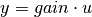Notes

This function is only useful for systems with invertible system matrix A.

All systems are first converted to state space form. The function then computes: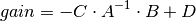Create a stable discrete random state space object.

Parameters: states: integer Number of state variables inputs: integer Number of system inputs outputs: integer Number of system outputs sys: StateSpace The randomly created linear system ValueError if any input is not a positive integer

Notes

If the number of states, inputs, or outputs is not specified, then the missing numbers are assumed to be 1. The poles of the returned system will always have a magnitude less than 1.

control.matlab.evalfr(sys, x)

Evaluate the transfer function of an LTI system for a single complex number x.

To evaluate at a frequency, enter x = omega*j, where omega is the frequency in radians

Parameters: sys: StateSpace or TransferFunction Linear system x: scalar Complex number fresp: ndarray

Notes

This function is a wrapper for StateSpace.evalfr and TransferFunction.evalfr.

Examples

>>> sys = ss("1. -2; 3. -4", "5.; 7", "6. 8", "9.")
>>> evalfr(sys, 1j)
array([[ 44.8-21.4j]])
>>> # This is the transfer function matrix evaluated at s = i.


Todo

control.matlab.frd(*args)

Construct a Frequency Response Data model, or convert a system

frd models store the (measured) frequency response of a system.

This function can be called in different ways:

frd(response, freqs)
Create an frd model with the given response data, in the form of complex response vector, at matching frequency freqs [in rad/s]
frd(sys, freqs)
Convert an LTI system into an frd model with data at frequencies freqs.
Parameters: response: array_like, or list complex vector with the system response freq: array_lik or lis vector with frequencies sys: LTI (StateSpace or TransferFunction) A linear system sys: FRD New frequency response system

ss, tf

control.matlab.freqresp(sys, omega)

Frequency response of an LTI system at multiple angular frequencies.

Parameters: sys: StateSpace or TransferFunction Linear system omega: array_like List of frequencies mag: ndarray phase: ndarray omega: list, tuple, or ndarray

Notes

This function is a wrapper for StateSpace.freqresp and TransferFunction.freqresp. The output omega is a sorted version of the input omega.

Examples

>>> sys = ss("1. -2; 3. -4", "5.; 7", "6. 8", "9.")
>>> mag, phase, omega = freqresp(sys, [0.1, 1., 10.])
>>> mag
array([[[ 58.8576682 ,  49.64876635,  13.40825927]]])
>>> phase
array([[[-0.05408304, -0.44563154, -0.66837155]]])


Todo

#>>> sys = rss(3, 2, 2) #>>> mag, phase, omega = freqresp(sys, [0.1, 1., 10.]) #>>> mag[0, 1, :] #array([ 55.43747231, 42.47766549, 1.97225895]) #>>> phase[1, 0, :] #array([-0.12611087, -1.14294316, 2.5764547 ]) #>>> # This is the magnitude of the frequency response from the 2nd #>>> # input to the 1st output, and the phase (in radians) of the #>>> # frequency response from the 1st input to the 2nd output, for #>>> # s = 0.1i, i, 10i.

control.matlab.impulse(sys, T=None, input=0, output=None)

Impulse response of a linear system

If the system has multiple inputs or outputs (MIMO), one input has to be selected for the simulation. Optionally, one output may be selected. If no selection is made for the output, all outputs are given. The parameters input and output do this. All other inputs are set to 0, all other outputs are ignored.

Parameters: sys: StateSpace, TransferFunction LTI system to simulate T: array-like object, optional Time vector (argument is autocomputed if not given) input: int Index of the input that will be used in this simulation. output: int Index of the output that will be used in this simulation. yout: array Response of the system T: array Time values of the output

Examples

>>> yout, T = impulse(sys, T)

control.matlab.initial(sys, T=None, X0=0.0, input=None, output=None)

Initial condition response of a linear system

If the system has multiple outputs (?IMO), optionally, one output may be selected. If no selection is made for the output, all outputs are given.

Parameters: sys: StateSpace, or TransferFunction LTI system to simulate T: array-like object, optional Time vector (argument is autocomputed if not given) X0: array-like object or number, optional Initial condition (default = 0) Numbers are converted to constant arrays with the correct shape. input: int This input is ignored, but present for compatibility with step and impulse. output: int If given, index of the output that is returned by this simulation. yout: array Response of the system T: array Time values of the output

Examples

>>> yout, T = initial(sys, T, X0)

control.matlab.lsim(sys, U=0.0, T=None, X0=0.0)

Simulate the output of a linear system.

As a convenience for parameters U, X0: Numbers (scalars) are converted to constant arrays with the correct shape. The correct shape is inferred from arguments sys and T.

Parameters: sys: LTI (StateSpace, or TransferFunction) LTI system to simulate U: array-like or number, optional Input array giving input at each time T (default = 0). If U is None or 0, a special algorithm is used. This special algorithm is faster than the general algorithm, which is used otherwise. T: array-like Time steps at which the input is defined, numbers must be (strictly monotonic) increasing. X0: array-like or number, optional Initial condition (default = 0). yout: array Response of the system. T: array Time values of the output. xout: array Time evolution of the state vector.

Examples

>>> yout, T, xout = lsim(sys, U, T, X0)

control.matlab.margin(*args)

Calculate gain and phase margins and associated crossover frequencies

Function margin takes either 1 or 3 parameters.

Parameters: sys : StateSpace or TransferFunction Linear SISO system mag, phase, w : array_like Input magnitude, phase (in deg.), and frequencies (rad/sec) from bode frequency response data gm, pm, Wcg, Wcp : float Gain margin gm, phase margin pm (in deg), gain crossover frequency (corresponding to phase margin) and phase crossover frequency (corresponding to gain margin), in rad/sec of SISO open-loop. If more than one crossover frequency is detected, returns the lowest corresponding margin.

Examples

>>> sys = tf(1, [1, 2, 1, 0])
>>> gm, pm, Wcg, Wcp = margin(sys)

control.matlab.ngrid()

Nichols chart grid

Plots a Nichols chart grid on the current axis, or creates a new chart if no plot already exists.

Parameters: cl_mags : array-like (dB), optional Array of closed-loop magnitudes defining the iso-gain lines on a custom Nichols chart. cl_phases : array-like (degrees), optional Array of closed-loop phases defining the iso-phase lines on a custom Nichols chart. Must be in the range -360 < cl_phases < 0 None
control.matlab.pole(sys)

Compute system poles.

Parameters: sys: StateSpace or TransferFunction Linear system poles: ndarray Array that contains the system’s poles. NotImplementedError when called on a TransferFunction object

zero

Notes

This function is a wrapper for StateSpace.pole and TransferFunction.pole.

control.matlab.rlocus(sys, klist=None, **keywords)

Root locus plot

The root-locus plot has a callback function that prints pole location, gain and damping to the Python consol on mouseclicks on the root-locus graph.

Parameters: sys: StateSpace or TransferFunction Linear system klist: iterable, optional optional list of gains xlim : control of x-axis range, normally with tuple, for other options, see matplotlib.axes ylim : control of y-axis range Plot : boolean (default = True) If True, plot magnitude and phase PrintGain: boolean (default = True) If True, report mouse clicks when close to the root-locus branches, calculate gain, damping and print rlist: list of roots for each gain klist: list of gains used to compute roots

Create a stable continuous random state space object.

Parameters: states: integer Number of state variables inputs: integer Number of system inputs outputs: integer Number of system outputs sys: StateSpace The randomly created linear system ValueError if any input is not a positive integer

Notes

If the number of states, inputs, or outputs is not specified, then the missing numbers are assumed to be 1. The poles of the returned system will always have a negative real part.

control.matlab.ss(*args)

Create a state space system.

The function accepts either 1, 4 or 5 parameters:

ss(sys)
Convert a linear system into space system form. Always creates a new system, even if sys is already a StateSpace object.
ss(A, B, C, D)

Create a state space system from the matrices of its state and output equations: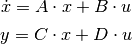ss(A, B, C, D, dt)

Create a discrete-time state space system from the matrices of its state and output equations: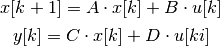The matrices can be given as array like data types or strings. Everything that the constructor of numpy.matrix accepts is permissible here too.

Parameters: sys: StateSpace or TransferFunction A linear system A: array_like or string System matrix B: array_like or string Control matrix C: array_like or string Output matrix D: array_like or string Feed forward matrix dt: If present, specifies the sampling period and a discrete time system is created out: StateSpace The new linear system ValueError if matrix sizes are not self-consistent

Examples

>>> # Create a StateSpace object from four "matrices".
>>> sys1 = ss("1. -2; 3. -4", "5.; 7", "6. 8", "9.")

>>> # Convert a TransferFunction to a StateSpace object.
>>> sys_tf = tf([2.], [1., 3])
>>> sys2 = ss(sys_tf)

control.matlab.ss2tf(*args)

Transform a state space system to a transfer function.

The function accepts either 1 or 4 parameters:

ss2tf(sys)
Convert a linear system into space system form. Always creates a new system, even if sys is already a StateSpace object.
ss2tf(A, B, C, D)

Create a state space system from the matrices of its state and output equations.

For details see: ss()

Parameters: sys: StateSpace A linear system A: array_like or string System matrix B: array_like or string Control matrix C: array_like or string Output matrix D: array_like or string Feedthrough matrix out: TransferFunction New linear system in transfer function form ValueError if matrix sizes are not self-consistent, or if an invalid number of arguments is passed in TypeError if sys is not a StateSpace object

Examples

>>> A = [[1., -2], [3, -4]]
>>> B = [[5.], ]
>>> C = [[6., 8]]
>>> D = [[9.]]
>>> sys1 = ss2tf(A, B, C, D)

>>> sys_ss = ss(A, B, C, D)
>>> sys2 = ss2tf(sys_ss)

control.matlab.ssdata(sys)

Return state space data objects for a system

Parameters: sys: LTI (StateSpace, or TransferFunction) LTI system whose data will be returned (A, B, C, D): list of matrices State space data for the system
control.matlab.step(sys, T=None, X0=0.0, input=0, output=None)

Step response of a linear system

If the system has multiple inputs or outputs (MIMO), one input has to be selected for the simulation. Optionally, one output may be selected. If no selection is made for the output, all outputs are given. The parameters input and output do this. All other inputs are set to 0, all other outputs are ignored.

Parameters: sys: StateSpace, or TransferFunction LTI system to simulate T: array-like object, optional Time vector (argument is autocomputed if not given) X0: array-like or number, optional Initial condition (default = 0) Numbers are converted to constant arrays with the correct shape. input: int Index of the input that will be used in this simulation. output: int If given, index of the output that is returned by this simulation. yout: array Response of the system T: array Time values of the output

Examples

>>> yout, T = step(sys, T, X0)

control.matlab.tf(*args)

Create a transfer function system. Can create MIMO systems.

The function accepts either 1 or 2 parameters:

tf(sys)
Convert a linear system into transfer function form. Always creates a new system, even if sys is already a TransferFunction object.
tf(num, den)

Create a transfer function system from its numerator and denominator polynomial coefficients.

If num and den are 1D array_like objects, the function creates a SISO system.

To create a MIMO system, num and den need to be 2D nested lists of array_like objects. (A 3 dimensional data structure in total.) (For details see note below.)

tf(num, den, dt)
Create a discrete time transfer function system; dt can either be a positive number indicating the sampling time or ‘True’ if no specific timebase is given.
Parameters: sys: LTI (StateSpace or TransferFunction) A linear system num: array_like, or list of list of array_like Polynomial coefficients of the numerator den: array_like, or list of list of array_like Polynomial coefficients of the denominator out: TransferFunction The new linear system ValueError if num and den have invalid or unequal dimensions TypeError if num or den are of incorrect type

Notes

num[i][j] contains the polynomial coefficients of the numerator for the transfer function from the (j+1)st input to the (i+1)st output. den[i][j] works the same way.

The list [2, 3, 4] denotes the polynomial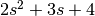.

Examples

>>> # Create a MIMO transfer function object
>>> # The transfer function from the 2nd input to the 1st output is
>>> # (3s + 4) / (6s^2 + 5s + 4).
>>> num = [[[1., 2.], [3., 4.]], [[5., 6.], [7., 8.]]]
>>> den = [[[9., 8., 7.], [6., 5., 4.]], [[3., 2., 1.], [-1., -2., -3.]]]
>>> sys1 = tf(num, den)

>>> # Convert a StateSpace to a TransferFunction object.
>>> sys_ss = ss("1. -2; 3. -4", "5.; 7", "6. 8", "9.")
>>> sys2 = tf(sys1)

control.matlab.tf2ss(*args)

Transform a transfer function to a state space system.

The function accepts either 1 or 2 parameters:

tf2ss(sys)
Convert a linear system into transfer function form. Always creates a new system, even if sys is already a TransferFunction object.
tf2ss(num, den)

Create a transfer function system from its numerator and denominator polynomial coefficients.

For details see: tf()

Parameters: sys: LTI (StateSpace or TransferFunction) A linear system num: array_like, or list of list of array_like Polynomial coefficients of the numerator den: array_like, or list of list of array_like Polynomial coefficients of the denominator out: StateSpace New linear system in state space form ValueError if num and den have invalid or unequal dimensions, or if an invalid number of arguments is passed in TypeError if num or den are of incorrect type, or if sys is not a TransferFunction object

Examples

>>> num = [[[1., 2.], [3., 4.]], [[5., 6.], [7., 8.]]]
>>> den = [[[9., 8., 7.], [6., 5., 4.]], [[3., 2., 1.], [-1., -2., -3.]]]
>>> sys1 = tf2ss(num, den)

>>> sys_tf = tf(num, den)
>>> sys2 = tf2ss(sys_tf)

control.matlab.tfdata(sys)

Return transfer function data objects for a system

Parameters: sys: LTI (StateSpace, or TransferFunction) LTI system whose data will be returned (num, den): numerator and denominator arrays Transfer function coefficients (SISO only)
control.matlab.zero(sys)

Compute system zeros.

Parameters: sys: StateSpace or TransferFunction Linear system zeros: ndarray Array that contains the system’s zeros. NotImplementedError when called on a TransferFunction object or a MIMO StateSpace object